Ex 12.2

Chapter 12 Class 10 Areas related to Circles
Serial order wise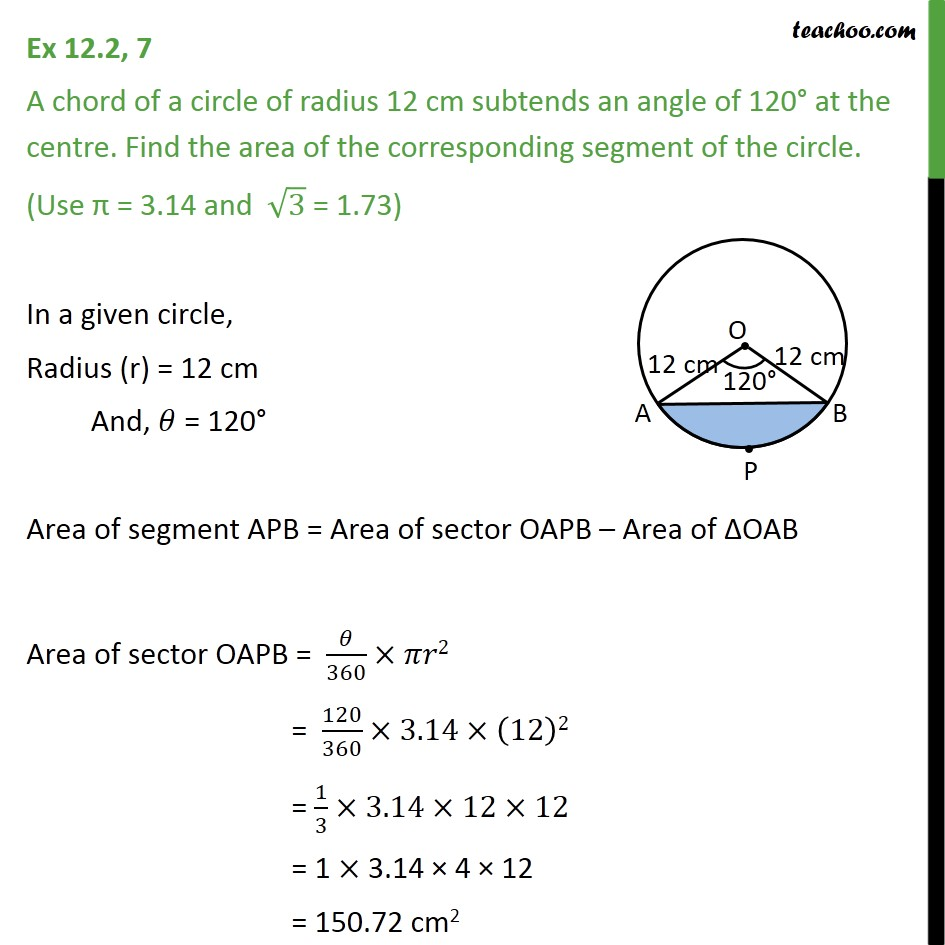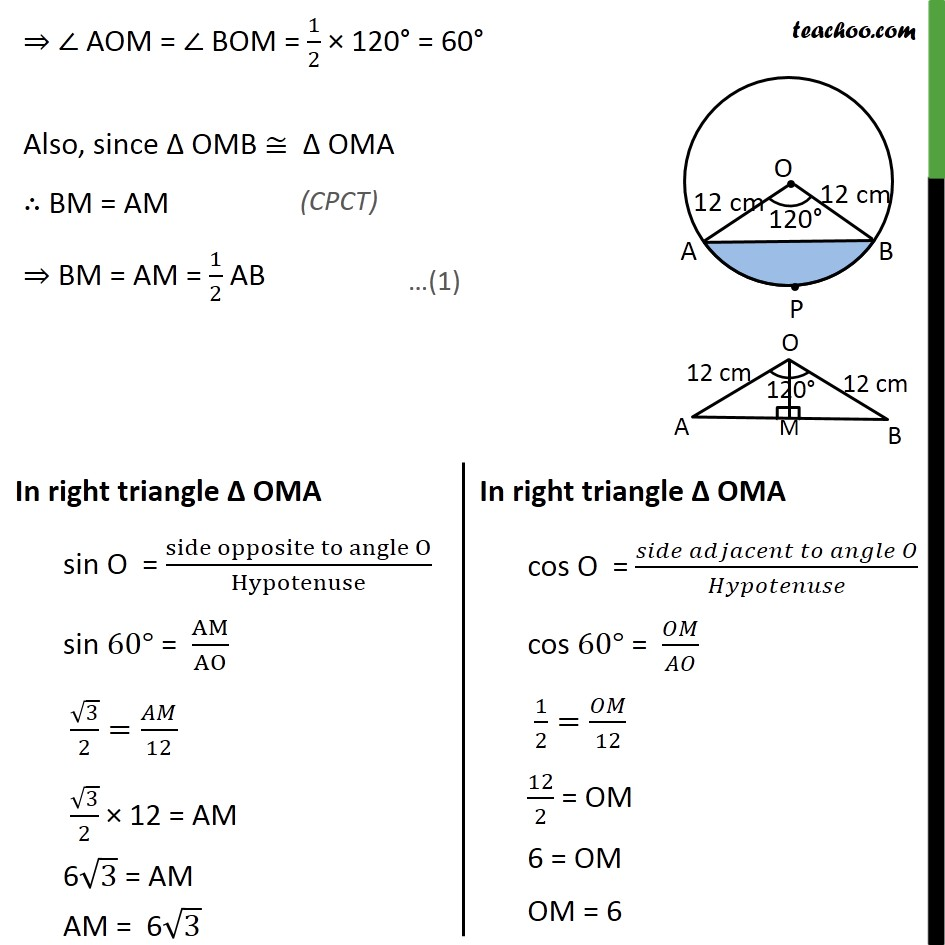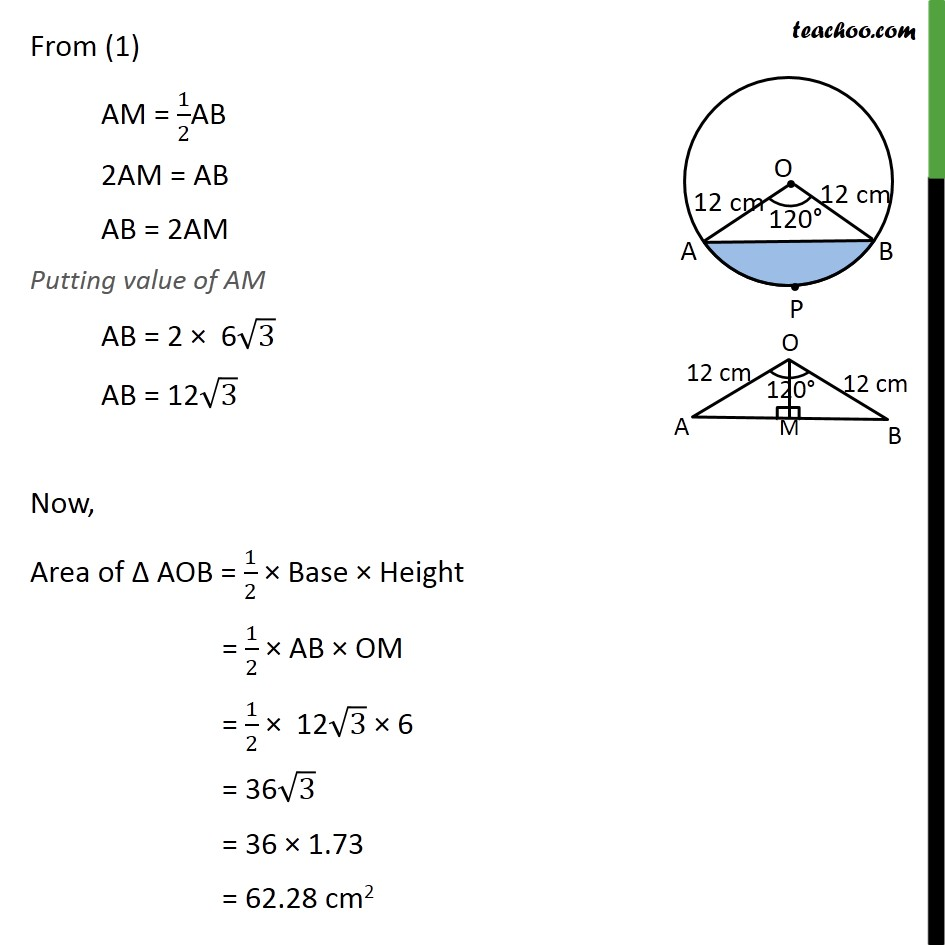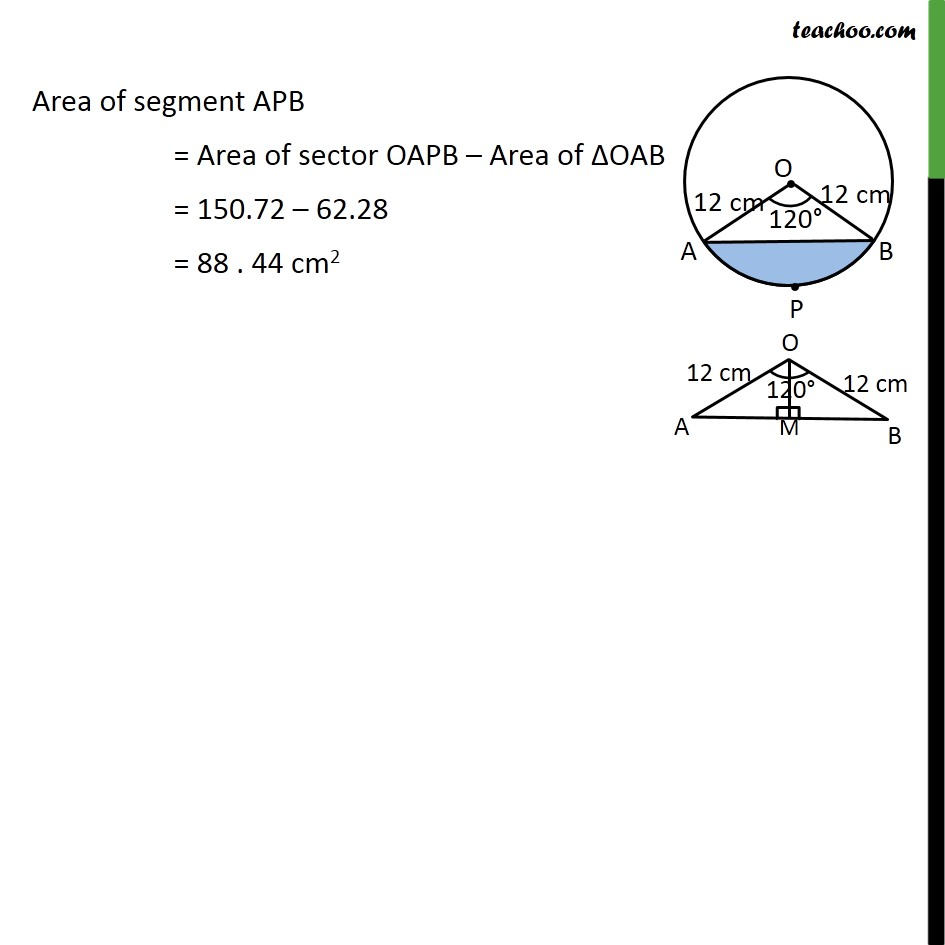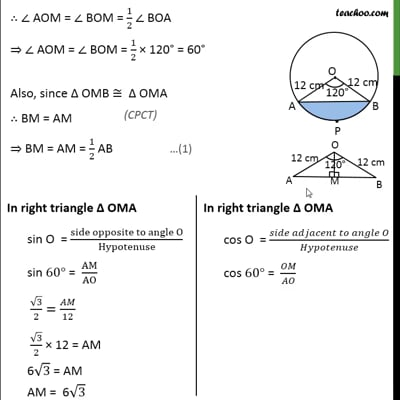This video is only available for Teachoo black users

Get live Maths 1-on-1 Classs - Class 6 to 12

### Transcript

Ex 12.2, 7 A chord of a circle of radius 12 cm subtends an angle of 120° at the centre. Find the area of the corresponding segment of the circle. (Use π = 3.14 and √3 = 1.73) In a given circle, Radius (r) = 12 cm And, 𝜃 = 120° Area of segment APB = Area of sector OAPB – Area of ΔOAB Area of sector OAPB = 𝜃/360×𝜋𝑟2 = 120/360×3.14×(12)2 = 1/3×3.14×12×12 = 1 × 3.14 × 4 × 12 = 150.72 cm2 Finding area of Δ AOB Area Δ AOB = 1/2 × Base × Height We draw OM ⊥ AB ∴ ∠ OMB = ∠ OMA = 90° In Δ OMA & Δ OMB ∠ OMA = ∠ OMB OA = OB OM = OM ∴ Δ OMA ≅ Δ OMB ⇒ ∠ AOM = ∠ BOM ∴ ∠ AOM = ∠ BOM = 1/2 ∠ BOA ⇒ ∠ AOM = ∠ BOM = 1/2 × 120° = 60° Also, since Δ OMB ≅ Δ OMA ∴ BM = AM ⇒ BM = AM = 1/2 AB From (1) AM = 1/2AB 2AM = AB AB = 2AM Putting value of AM AB = 2 × 6√3 AB = 12√3 Now, Area of Δ AOB = 1/2 × Base × Height = 1/2 × AB × OM = 1/2 × 12√3 × 6 = 36√3 = 36 × 1.73 = 62.28 cm2 Area of segment APB = Area of sector OAPB – Area of ΔOAB = 150.72 – 62.28 = 88 . 44 cm2